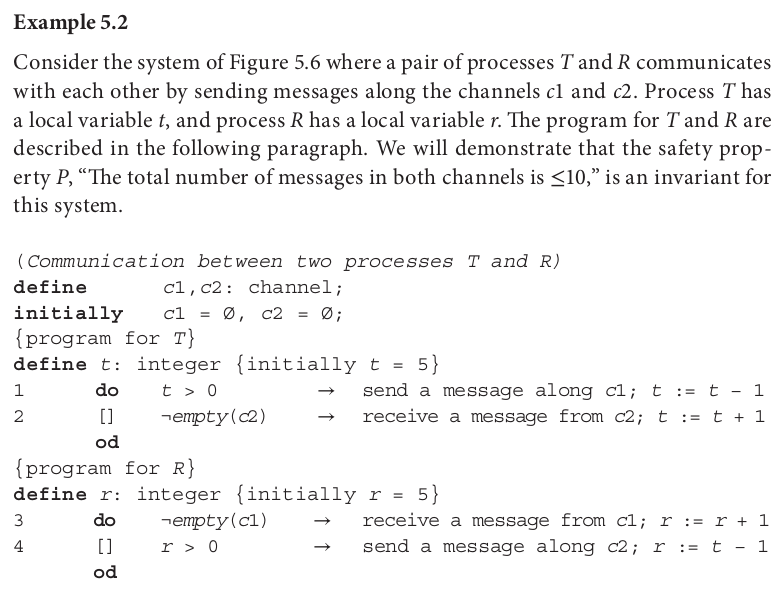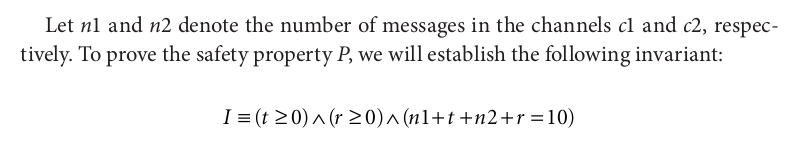December 2, 2018

# Coq Proof of Safety for a Two Node Bidirectional Network

In Sukumar Ghosh’s book Distributed Systems: An Algorithmic Approach, an example of a safety property proof is given for a simple network consisting of two nodes communicating bidirectionally via two unidirectional channels. Here I’ll walk through how Ghosh’s informal proof can be translated into a formal proof using Coq.

On page 93, Ghosh poses Example 5.2 as follows:We can easily define this network in Coq, then prove that the safety property P does indeed hold.

## Network Definition

We begin by importing various modules and opening various scopes:

``````Require Import List String Omega ZArith.

Local Open Scope string_scope.
Local Open Scope list_scope.
Local Open Scope Z_scope.
``````

Then we define a type `channel` to be a list of strings, without elaborating any structure that those strings should have:

``````Definition channel : Type := list string.
``````

Each message will simply be the string literal “Message.”:

``````Definition msg : string := " ""Message."" ".
``````

Next we need some kind of structure that describes the state of the network, which is comprised of four entities: local variable `t`, local variable `r`, channel `c1`, and channel `c2`. There are various ways to do this, but I chose to use Coq’s `Record` macro, which generates an `Inductive` behind the scenes along with accessor functions corresponding to each entity within:

``````Record netstate := NetState {t : Z; r : Z; c1 : channel; c2 : channel}.
``````

Now we can define the network’s initial state as described by Ghosh: both local variables are initialized to five, and both channels are empty.

``````Definition initial := {| t := 5 ; r := 5 ; c1 := nil ; c2 := nil |}.
``````

## Network Behavior as an Inductive Relation

With the structure of the network defined, we now need a definition for how the network behaves. We can create one using an inductive relation:

``````Inductive netEvalR : netstate -> netstate -> Prop :=
``````

First is the initial state, which is trivially related to itself:

``````| Initial : (netEvalR initial initial)
``````

Next is step 1 as Ghosh defines it: if local variable `t` is greater than 0, decrement `t` and send a message on channel `c1`. Note that we must be more explicit by adding the requirement that the current state `st'` must be related to a previous state `st` by the evaluation relation: `netEvalR st st' ->`. Without this requirement, the relation would hold for states that aren’t reachable from the initial state of the network, which we do not want. In fact, omitting this requirement would make it impossible to write a proof of safety, as our proof will rely on induction and we will need specific constraints on preceding network states that can be used to show that safety properties will carry though to suceeding states.

``````| Step1 : forall st st',
netEvalR st st' ->
st'.(t) > 0 ->
(netEvalR st' {| t := st'.(t) - 1 ; r := st'.(r) ;
c1 := msg :: st'.(c1) ; c2 := st'.(c2) |})
``````

The other three states proceed similarly, just as Ghosh defines them above:

``````| Step2 : forall st st',
netEvalR st st' ->
st'.(c2) <> nil ->
(netEvalR st' {| t := st'.(t) + 1 ; r := st'.(r) ;
c1 := st'.(c1) ; c2 := (tl st'.(c2)) |})
| Step3 : forall st st',
netEvalR st st' ->
st'.(c1) <> nil ->
(netEvalR st' {| t := st'.(t) ; r := st'.(r) + 1 ;
c1 := (tl st'.(c1)) ; c2 := st'.(c2) |})
| Step4 : forall st st',
netEvalR st st' ->
st'.(r) > 0 ->
(netEvalR st' {| t := st'.(t) ; r := st'.(r) - 1 ;
c1 := st'.(c1) ; c2 := (msg :: st'.(c2)) |}).
``````

## Safety Property, Part 1

Here’s how Ghosh defines the desired safety property for this system:I’m going to break this in half for ease of proving. The first half consists of the first two terms of Ghosh’s invariant `I`, which describe the local variables `t` and `r`:

``````Definition safety1 (st' : netstate) : Prop := st'.(t) >= 0 /\ st'.(r) >= 0.
``````

We’ll now prove that this proposition holds for all network states `st'` reachable from all previous network states `st`:

``````Lemma safety1_holds: forall st st' : netstate,
(netEvalR st st') ->
safety1 st'.
``````

We start by introducing variables and hypotheses, then splitting the proposition into its respective halves:

``````Proof.
intros st st' H. split.
``````

The first half asserts that local variable `t` is non-negative. We proceed by induction on hypothesis `H`, which assumes that `st` and `st'` are related network states. Induction gives us five subgoals, one for each possible step of the evaluation relation:

1. In the initial state, local variable `t` is 5, which is of course >= 0. Use `omega` to dispatch this subgoal.
2. In step 1, `t` is decremented by 1. Since `t` is strictly > 0 in the preceding step of the relation as a condition of step 1 (represented as hypothesis `H0` in the context of the proof), we know that after the decrement `t` will be >= 0. Use `omega` to dispatch.
3. In step 2, `t` is incremented by 1. By the induction hypothesis, `t` is >= 0 prior to the increment, so of course `t` is >= 0 after the increment as well. Use `omega` to dispatch.
4. In step 3, `t` is unchanged. By the induction hypothesis we assume that `t` is greater than or equal to 0 in the previous network state, so the same holds when `t` is unchanged. Use `omega` or `assumption` to dispatch.
5. In step 4, `t` is unchanged as in step 3. Use `omega` or `assumption` to dispatch.

The same use of induction followed by `omega` on all of the subgoals also suffices to prove the second half of the proposition asserting `r` is non-negative. Putting it all together, the proof is:

``````Lemma safety1_holds: forall st st' : netstate,
(netEvalR st st') ->
safety1 st'.
Proof.
intros st st' H. split; induction H; simpl; omega.
Qed.
``````

## Safety Property, Part 2

Now we’ll prove the second half of Ghosh’s invariant `I` above, defining it in Coq as follows:

``````Definition safety2 (st' : netstate) : Prop :=
Zlength st'.(c1) + Zlength st'.(c2) + st'.(t) + st'.(r) = 10.
``````

In other words, we want to prove that no matter what state the network is in, the sum of local variable `t`, local variable `r` and the lengths of channels `c1` and `c2` is always 10.

We’ll now prove that this proposition holds for all network states `st'` reachable from all previous network states `st`:

``````Lemma safety2_holds : forall st st' : netstate,
(netEvalR st st') ->
safety2 st'.
``````

After some basic setup, we proceed by induction on hypothesis `H`:

``````Proof.
intros st st' H. unfold safety2. induction H; simpl.
``````

As above, induction generates five subgoals, one for each step of the evaluation relation:

1. In the initial state of the network, local variables `t` and `r` are 5, while the lengths of the channels are each 0, thus `safety1` trivially holds (use `reflexivity`).
2. In step 1 of the relation, a single message is appended to channel `c1` concommitant with a decrement to the value of `t`. First we `rewrite Zlength_cons` to “extract” the implied addition, then `omega` will cancel it out with the decrement, giving us a goal that matches our induction hypothesis.
3. In step 2 of the relation, a message is removed from channel `c2` concommitant with an increment to the value of `t`. Although it’s obvious to us that these cancel out, we have to do some work to show that the length of `c2` will always decrease by one. To do so, `destruct (c2 st')` to get two subgoals:
• The first subgoal represents the case of an empty `c2`. But we know this is impossible because a condition of step 2 requires that `c2` not be nil. Dispatch with `contradiction`.
• The second subgoals represents a `c2` containing at least one message. The tail of a list `(s :: c)` is simply c, which we get with `simpl`. Then we `rewrite Zlength_cons in IHnetEvalR` to extract the implied addition, which matches up with the increment of `t` in the goal, and `omega` will do the rest.
4. The flow for step 3 of the relation is the same as that for step 3, only with `(c1 st')` destructed instead of `(c2 st')`.
5. The flow for step 4 of the relation is exactly the same as that for step 1.

The resultant proof that `safety2` holds is therefore:

``````Lemma safety2_holds : forall st st' : netstate,
(netEvalR st st') ->
safety2 st'.
Proof.
intros st st' H. unfold safety2. induction H; simpl;
try reflexivity;                           (* Initial state *)
try (rewrite Zlength_cons; omega);         (* Steps 1 and 4 *)
[destruct (c2 st') | destruct (c1 st')];   (* Steps 2 and 3 *)
(simpl; rewrite Zlength_cons in IHnetEvalR; omega).
Qed.
``````

## Concluding Theorem

The preceding lemmas encapsulate the work required to formally prove that Ghosh’s safety invariant holds for all reachable network states. We can now combine them into a succint concluding theorem:

``````Theorem safety_holds : forall st st' : netstate,
(netEvalR st st') -> safety1 st' /\ safety2 st'.
Proof.
intros st st' H. split;
[apply (safety1_holds st st') | apply (safety2_holds st st')];
assumption.
Qed.
``````